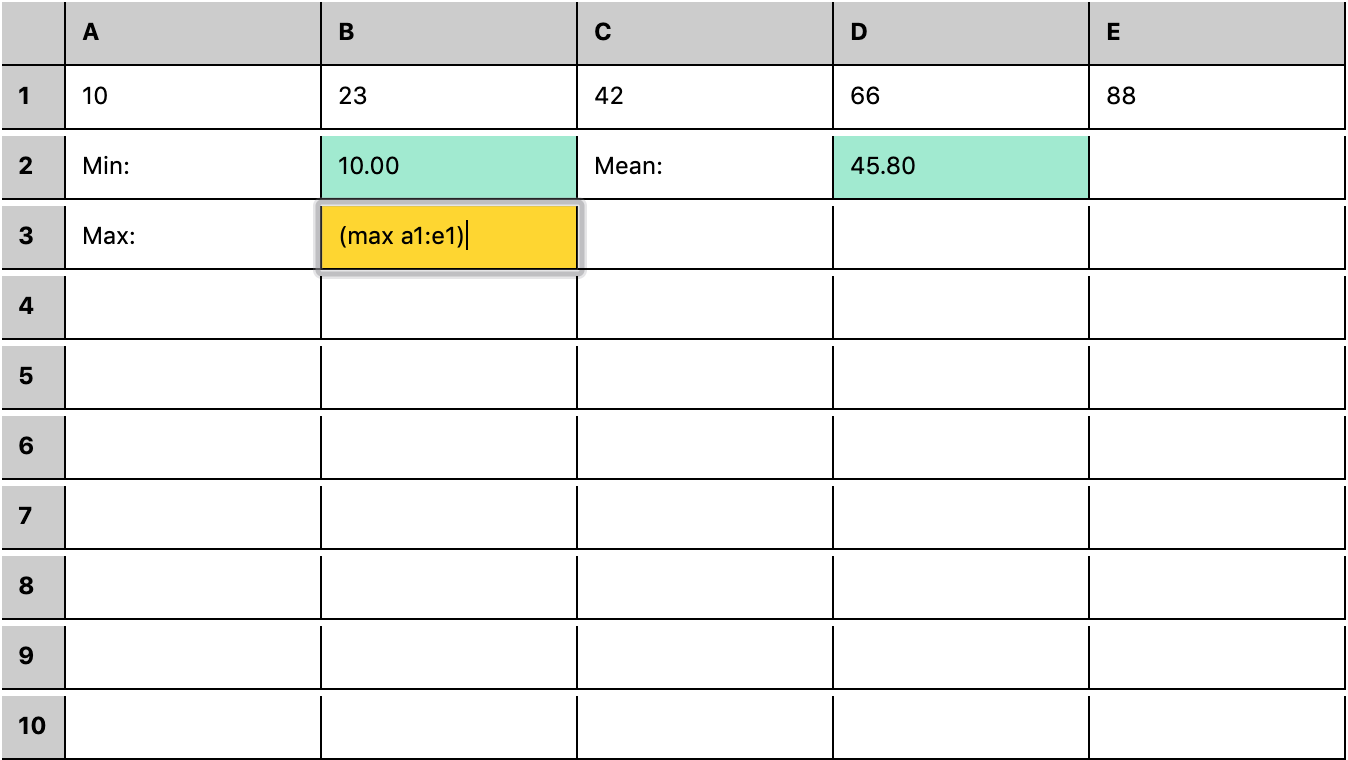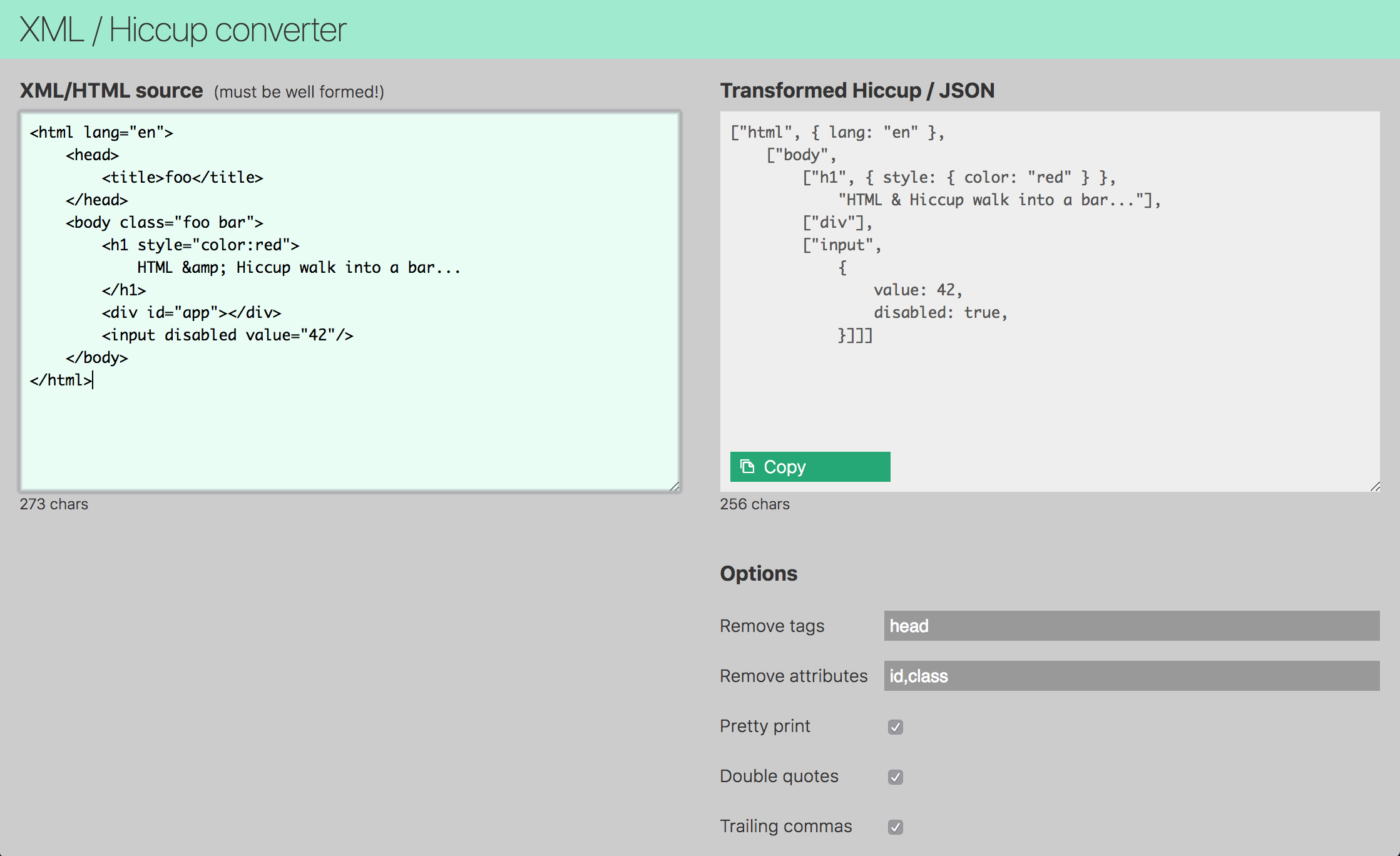next() Packaged Middleware

# npm

## @thi.ng/defmulti1.3.13 • Public • Published

#This project is part of the @thi.ng/umbrella monorepo.

Dynamically extensible multiple dispatch via user supplied dispatch function, with minimal overhead and support for dispatch value inheritance hierarchies (more flexible and independent of any actual JS type relationships).

### Status

STABLE - used in production

Search or submit any issues for this package

## Installation

yarn add @thi.ng/defmulti
// ES module
<script type="module" src="https://unpkg.com/@thi.ng/defmulti?module" crossorigin></script>

// UMD
<script src="https://unpkg.com/@thi.ng/defmulti/lib/index.umd.js" crossorigin></script>

Package sizes (gzipped, pre-treeshake): ESM: 801 bytes / CJS: 863 bytes / UMD: 939 bytes

## Usage examples

Several demos in this repo's /examples directory are using this package.

A selection:

Screenshot Description Live demo Sourcerstream based spreadsheet w/ S-expression formula DSL Demo SourceXML/HTML/SVG to hiccup/JS conversion Demo Source

## API

Generated API docs

### defmulti()

defmulti returns a new multi-dispatch function using the provided dispatcher function. The dispatcher acts as a mapping function, can take any number of arguments and must produce a dispatch value (string, number or symbol) used to lookup an implementation. If found, the impl is called with the same args. If no matching implementation is available, attempts to dispatch to DEFAULT impl. If none is registered, an error is thrown.

defmulti provides generics for type checking up to 8 args (plus the return type) and the generics will also apply to all implementations. If more than 8 args are required, defmulti will fall back to an untyped varargs solution.

The function returned by defmulti can be called like any other function, but also exposes the following operations:

• .add(id, fn) - adds/overrides implementation for given dispatch value
• .remove(id) - removes implementation for dispatch value
• .callable(...args) - takes same args as if calling the multi-function, but only checks if an implementation exists for the given args. Returns boolean.
• .isa(child, parent) - establish dispatch value relationship hierarchy
• .impls() - returns set of all dispatch values which have an implementation
• .rels() - return all dispatch value relationships
• .parents(id) - direct parents of dispatch value id
• .ancestors(id) - transitive parents of dispatch value id
• .dependencies() - returns iterator of all dispatch value relationship pairs

#### Dispatch value hierarchies

To avoid code duplication, dispatch values can be associated in child-parent relationships and implementations only defined for some ancestors. Iff no implementation exists for a concrete dispatch value, defmulti first attempts to find an implementation for any ancestor dispatch value before using the DEFAULT implementation.

These relationships can be defined via an additional (optional) object arg to defmulti and/or dynamically extended via the .isa(child, parent) call to the multi-function. Relationships can also be queried via .parents(id) and .ancestors(id).

Note: If multiple direct parents are defined for a dispatch value, then it's currently undefined which implementation will be picked. If this causes issues to people, parents could be implemented as sorted list (each parent with weight) instead of Sets, but this will have perf impact... please open an issue if you run into problems!

const foo = defmulti((x )=> x);
foo.isa(23, "odd");
foo.isa(42, "even");
foo.isa("odd", "number");
foo.isa("even", "number");

foo.parents(23); // Set { "odd" }
foo.ancestors(23); // Set { "odd", "number" }

foo.parents(1); // undefined
foo.ancestors(1); // Set { }

foo.add("odd", (x) => ${x} is odd); foo.add("number", (x) => ${x} is a number);

// dispatch values w/ implementations
foo.impls();
// Set { "odd", "even", "number", "23", "42" }

foo(23); // "23 is odd"
foo(42); // "42 is a number"
foo(1);  // error (missing impl & no default)

foo.callable(1) // false

Same example, but with relationships provided as argument to defmulti:

const foo = defmulti((x) => x, {
23: ["odd"],
42: ["even"],
"odd": ["number"],
"even": ["number"],
});

foo.rels();
// { "23": Set { "odd" },
//   "42": Set { "even" },
//   odd: Set { "number" },
//   even: Set { "number" } }

### implementations()

Syntax-sugar intended for sets of multi-methods sharing same dispatch values / logic. Takes a dispatch value, an object of "is-a" relationships and a number of multi-methods, each with an implementation for the given dispatch value.

The relations object has dispatch values (parents) as keys and arrays of multi-methods as their values. For each multi-method associates the given type with the related parent dispatch value to delegate to its implementation (see .isa() above).

The remaining implementations are associated with their related multi-method and the given type dispatch value.

foo = defmulti((x) => x.id);
bar = defmulti((x) => x.id);
bax = defmulti((x) => x.id);
baz = defmulti((x) => x.id);

// define impls for dispatch value a
implementations(
"a",

// delegate bax & baz impls to dispatch val b
{
b: [bax, baz]
},

// concrete multi-fn impls
foo,
(x) => foo: ${x.val}, bar, (x) => bar:${x.val.toUpperCase()}
);

// some parent impls for bax & baz
bax.add("b", (x) => bax: ${x.id}); baz.add("c", (x) => baz:${x.id});

// delegate to use "c" impl for "b"
baz.isa("b", "c");

foo({ id: "a", val: "alice" }); // "foo: alice"
bar({ id: "a", val: "alice" }); // "bar: ALICE"
bax({ id: "a", val: "alice" }); // "bax: a"
baz({ id: "a", val: "alice" }); // "baz: a"

baz.impls(); // Set { "c", "a", "b" }

Also see the WIP package @thi.ng/geom for a concreate realworld usage example.

### defmultiN()

Returns a multi-dispatch function which delegates to one of the provided implementations, based on the arity (number of args) when the function is called. Internally uses defmulti, so new arities can be dynamically added (or removed) at a later time. If no fallback is provided, defmultiN also registers a DEFAULT implementation which simply throws an IllegalArityError when invoked.

Note: Unlike defmulti no argument type checking is supported, however you can specify the return type for the generated function.

const foo = defmultiN<string>({
0: () => "zero",
1: (x) => one: ${x}, 3: (x, y, z) => three:${x}, ${y},${z}
});

foo();
// zero
foo(23);
// one: 23
foo(1, 2, 3);
// three: 1, 2, 3
foo(1, 2);
// Error: illegal arity: 2

## Usage examples

import { defmulti, DEFAULT } from "@thi.ng/defmulti";

const visit = defmulti<any, void>((x) => Object.prototype.toString.call(x));

// register implementations for different dispatch types
// each dispatch value can only be registered once
visit.add("[object Object]", (x) => { for(let k in x) visit([k, x[k]]); });
// ignore null values
visit.add("[object Null]", (x) => { });
// DEFAULT matches all other dispatch values

// call like normal fn
visit([{a: 1, b: ["foo", "bar", null, 42]}])
// a
// 1
// b
// foo
// bar
// 42

See /test/index.ts for a variation of this example.

#### Dynamic dispatch: Simple S-expression interpreter

const exec = defmulti((x) => Array.isArray(x) ? x : typeof x);
exec.add("+", ([_, ...args]) => args.reduce((acc, n) => acc + exec(n), 0));
exec.add("*", ([_, ...args]) => args.reduce((acc, n) => acc * exec(n), 1));
exec.add(DEFAULT, (x) => { throw new Error(invalid expr: ${x}); }); // 10 * (1 + 2 + 3) + 6 exec(["+", ["*", 10, ["+", 1, 2, 3]], 6]); // 66 #### True multiple arg dispatch // interest rate calculator based on account type & balance thresholds const apr = defmulti( ({type, balance}) => ${type}-${balance < 1e4 ? "low" : balance < 5e4 ? "med" : "high"} ); apr.add("current-low", ({ balance }) => balance * 0.005); apr.add("current-med", ({ balance }) => balance * 0.01); apr.add("current-high", ({ balance }) => balance * 0.01); apr.add("savings-low", ({ balance }) => balance * 0.01); apr.add("savings-med", ({ balance }) => balance * 0.025); apr.add("savings-high", ({ balance }) => balance * 0.035); apr.add(DEFAULT, (x) => { throw new Error(invalid account type:${x.type})});

apr({type: "current", balance: 5000});
// 25
apr({type: "current", balance: 10000});
// 100
apr({type: "savings", balance: 10000});
// 250
apr({type: "isa", balance: 10000});
// Error: invalid account type: isa

#### Dispatch value graph visualization

To facilitate better introspection of dynamically constructed/added defmulti() implementations (with possibly deep hierarchies of dispatch values), we can utilize the .dependencies() method to extract all dispatch value relationships and use these to build dependency graph, which then can also be visualized.

import { defDGraph } from "@thi.ng/dgraph";
import { toDot } from "@thi.ng/dgraph-dot";

const fn = defmulti((x) => x);

// dummy impls

// dispatch value relationships
fn.isa("b", "a");
fn.isa("c", "b");
fn.isa("e", "d");

// build dependency graph and export as Graphviz DOT format
console.log(toDot(defDGraph(fn.dependencies()), { id: (id) => id }));
// digraph g {
// "b"[label="b"];
// "c"[label="c"];
// "e"[label="e"];
// "a"[label="a"];
// "d"[label="d"];
// "b" -> "a";
// "c" -> "b";
// "e" -> "d";
// }## Authors

Karsten Schmidt

If this project contributes to an academic publication, please cite it as:

@misc{thing-defmulti,
title = "@thi.ng/defmulti",
author = "Karsten Schmidt",
note = "https://thi.ng/defmulti",
year = 2018
}

## Keywords

### Install

npm i @thi.ng/defmulti

### Repository

github.com/thi-ng/umbrella

586

1.3.13